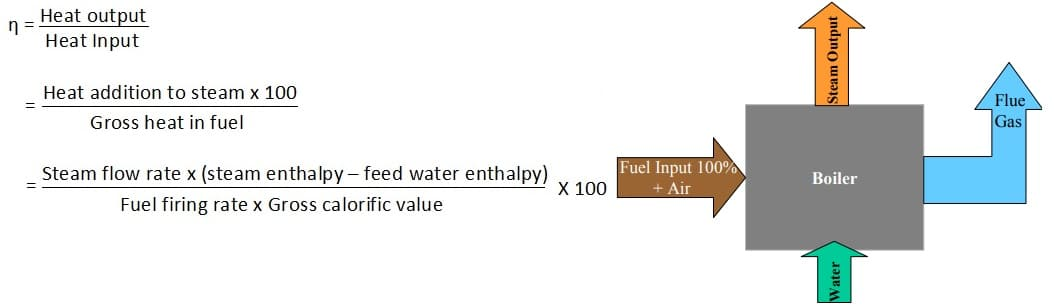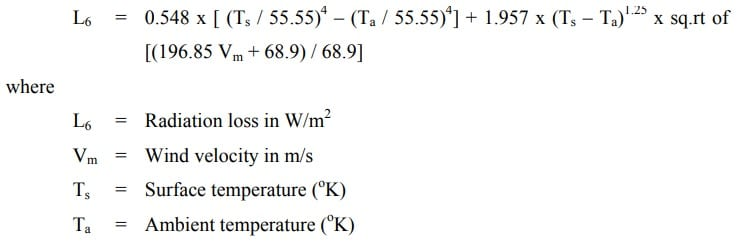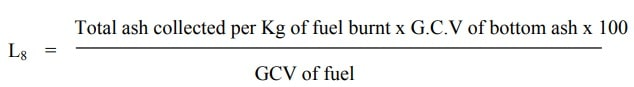# Boiler Efficiency

It is the ratio of heap output to heap Input.

Boiler Efficiency, η = Heat output / Heat Input = Heat in steam output (Kcals) / Heat in Fuel Input (Kcals)

## Reference Standards to Calculate Boiler Efficiency

1. The British Standard BS845: 1987

It describes the methods and conditions under which a boiler should be tested to determine its efficiency. For the testing to be done, the boiler should be operated under steady load conditions (generally full load) for a period of one hour after which readings would be taken during the next hour of steady operation to enable the efficiency to be calculated.

1. USA Standard is ‘ASME PTC-4-1

This includes both the direct and indirect methods

Most standards for computation of boiler efficiency, including IS 8753 and BS845 are designed for spot measurement of boiler efficiency. Invariably, all this standard does not includes blow down loss in the Efficiency determination process.

## Methods of Measuring Boiler Efficiency

There are two methods of measuring boiler efficiency:

• The Direct Method

In this method the energy gain of the working fluid (water and steam) is compared with the energy content of the boiler fuel.

• The Indirect Method

The efficiency is the difference between the losses and the energy input.

## Calculating Boiler Efficiency by Direct Method

This is also known as ‘input output method’ due to the fact that it needs only the useful output (steam) and the heat input (i.e. fuel) for evaluating the efficiency. This efficiency can be evaluated using the formula:1. Plant people can evaluate quickly the efficiency of boilers.
2. Requires few parameters for computation.
3. Needs few instruments for monitoring Demerits.

1. Does not give clues to the operator as to why efficiency of system is lower.
2. Does not calculate various losses accountable for various efficiency levels.
3. Evaporation ratio may mislead, if the steam is highly wet due to water carryover.

## Calculating Boiler Efficiency by Indirect Method

In this method efficiency is measured by measuring all the losses occurring in the boilers using the principles to be described. The disadvantages of the direct method can be overcome by this method, which calculates the various heat losses associated with boiler. The efficiency can be arrived at, by subtracting the heat loss fractions from 100. The various heat losses occurring in the boiler are:

The following losses are applicable to liquid, gas and solid fired boiler

### L6- Loss due to surface radiation and convection heat lossThe following losses are applicable to solid fuel fired boiler in addition to above

### L8- Unburnt losses in bottom ash (Carbon)Efficiency by indirect method = 100 – (L1+L2+L3+L4+L5+L6+L7+L8)

RELATED SEARCHES:

Boiler in thermal Power Plant